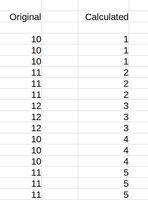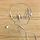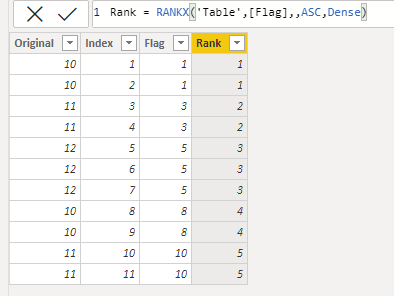cancel
Showing results for
Did you mean:Frequent Visitor

## Calculated Index column based on groups

Can anyone help me with this.?I need make the calculated column on the right hand side that only increments when the original field changes its value.

Noticed that the increments should keep increasing even though the a original group value may repeat the index should not.

Is there an option or some dax etc to achieve this?

1 ACCEPTED SOLUTIONCommunity Support

@fraza

This is logically not possible since you don't have a field to group by. As the workaround, you could add an index column and flag column before getting the rank, which is a bit confusing.

1. add an index column in power query

2. create a flag column

Flag =
var current_=[Original]
var current_1= CALCULATE(MAX([Original]),FILTER('Table',[Index]=EARLIER([Index])-1))
var current_2 = CALCULATE(MAX([Original]),FILTER('Table',[Index]=EARLIER([Index])-2))
var index_1=CALCULATE(SUM('Table'[Index]),FILTER('Table',[Index]=EARLIER([Index])-1))
var index_2=CALCULATE(SUM('Table'[Index]),FILTER('Table',[Index]=EARLIER([Index])-2))
Return
IF(current_=current_1&&current_1=current_2,index_2,IF(current_=current_1,index_1,[Index]))

3. create the rank columnPaul Zheng _ Community Support Team
If this post helps, please Accept it as the solution to help the other members find it more quickly.Community Support

@fraza

This is logically not possible since you don't have a field to group by. As the workaround, you could add an index column and flag column before getting the rank, which is a bit confusing.

1. add an index column in power query

2. create a flag column

Flag =
var current_=[Original]
var current_1= CALCULATE(MAX([Original]),FILTER('Table',[Index]=EARLIER([Index])-1))
var current_2 = CALCULATE(MAX([Original]),FILTER('Table',[Index]=EARLIER([Index])-2))
var index_1=CALCULATE(SUM('Table'[Index]),FILTER('Table',[Index]=EARLIER([Index])-1))
var index_2=CALCULATE(SUM('Table'[Index]),FILTER('Table',[Index]=EARLIER([Index])-2))
Return
IF(current_=current_1&&current_1=current_2,index_2,IF(current_=current_1,index_1,[Index]))

3. create the rank columnPaul Zheng _ Community Support Team
If this post helps, please Accept it as the solution to help the other members find it more quickly.